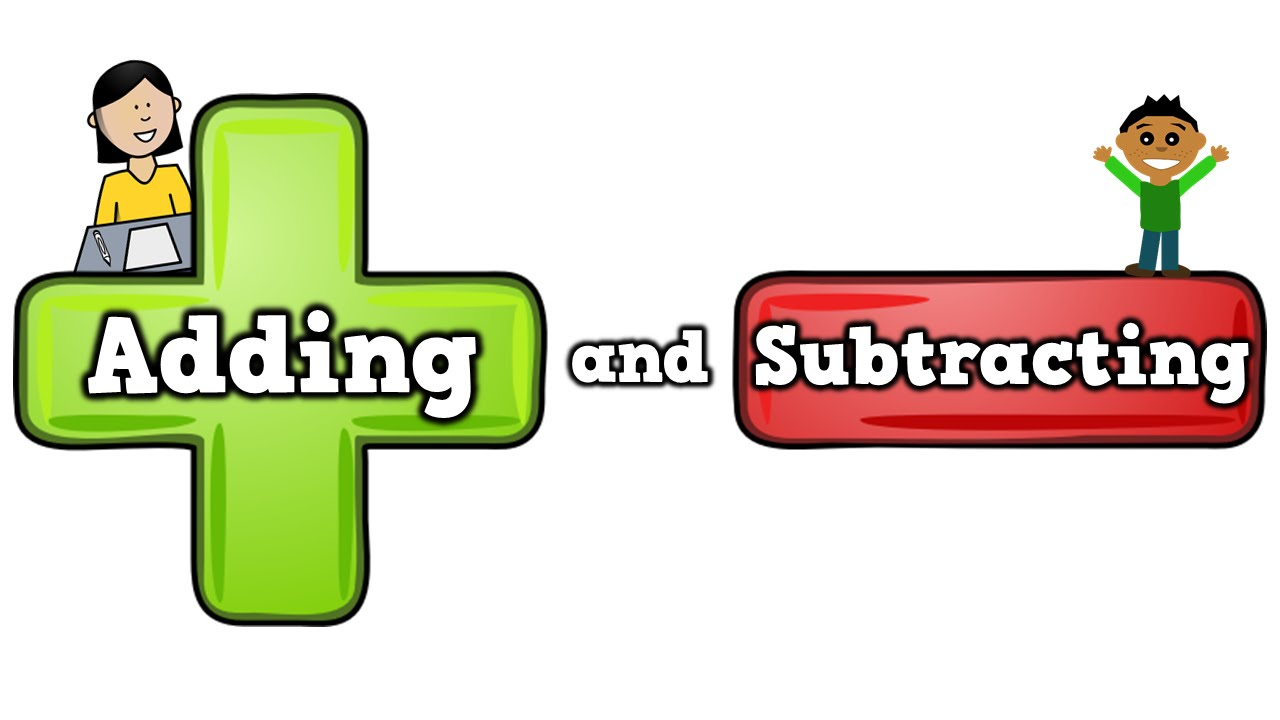This article explains the process of addition and subtraction of numbers. A mathematical equation has numbers and symbols in the place of words. The different set of numbers include:

• Natural Numbers (N) – (counting numbers) – {1, 2, 3, 4, 5, …}
• Whole Numbers (W) – {0, 1, 2, 3, 4, 5, …}
• Real numbers (R) – the numbers that can be written as a decimal or a fraction.
• Integers (Z) – The set of all whole numbers plus all its negatives of the natural numbers.
• Rational numbers (Q) – All fractions where the numerator and denominator are integer numbers.

Addition and subtraction of numbers involve the operations with the same and different sign.

• 3 + 3 = 6
• 3 + (- 3) = 0
• – 3 + (- 3) = – 6
• – 3 – (- 3) = 0

A number line can be used to represent the addition and subtraction of numbers.24 + 15 = 39

The above are the ways of working with numbers. It can be termed as arithmetical operations. The term “sum” is used to find the resultant number when two numbers are added together and “difference” when two numbers are subtracted. Addition and subtraction of numbers can be applied to rational numbers and irrational numbers, real numbers, complex numbers also. The addition and subtraction of algebraic expressions involves the same rules.

A fraction consists of a numerator and denominator where the numerator represents “part of the whole” and the denominator is the “whole”. The term fraction originates from the Latin word “fractus” which means broken. There are two types of fractions namely like and unlike fractions. The fractions where the denominators are the same are called like fractions whereas those with different denominators are unlike fractions. The method of rationalisation can be used while adding unlike and like fractions.

The below process can be followed while adding and subtracting fractions with same and different denominators.

When the denominators are the same, numerators are added and the denominator is retained.

For example: (a / d) + (b / d) = [a + b] / d

While subtracting fractions with same denominators, subtract the numerators and write the denominator as it is.

For example: (a / d) – (b / d) = [a – b] / d

The above actions can be taken while adding fractions with same denominators. In the case of addition of fractions with different denominators, LCM of denominators are taken by rationalising the denominator. The numerators and denominators of fractions are multiplied in such a way that the denominator of both fractions becomes equal to the value of LCM. After this, the numerators are added together keeping the common denominator. The method of addition of fraction with different denominators can be understood by the below example.

Add: 3 / 12 + 5 / 2

### Solution:

The given fraction is reduced to a simplified form:

3 / 12 can be reduced to 1 / 4 as 3 times 4 gives 12. The fraction now becomes (1 / 4) + 5 / 2.

The denominators of the two fractions are not the same, i.e. 4 and 2.

Step 1: The denominators must be rationalised by taking the LCM.

LCM of 4 and 2 = 2

Step 2: The second fraction is multiplied and divided by 2 such that the denominator is equal to 4.

(5 / 2) x (2 / 2) = 10 / 4

Step 3: As we can see that the denominators are the same for both the fractions, hence they can be added.

(1 / 4) + (10 / 4)

Step 4: The numerators are added keeping the common denominator.

(1 + 10) / 4

= 11 / 4

[3 / 12] + [5 / 2] is 11 / 4.

To obtain more problems on the above topic, download BYJU’S – The Learning App from Google Play Store and watch interactive videos.